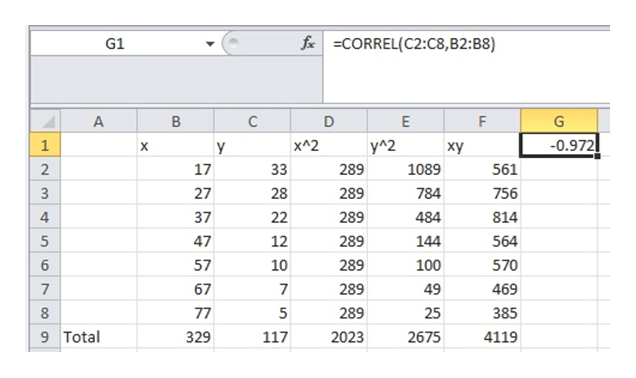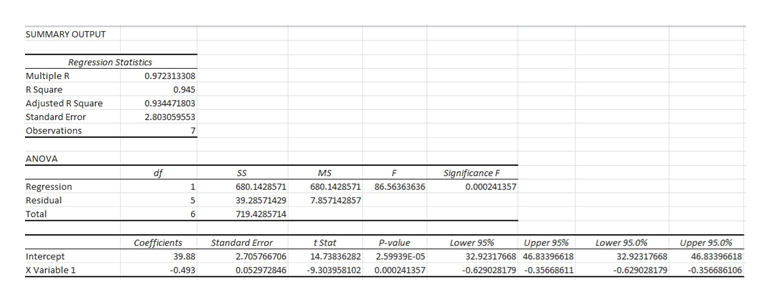Please answer the whole question. (1) Let x be the age in years of a licensed automobile driver. Let y be the percentage of all fatal accidents (for a given age) due to speeding. For example, the first data pair indicates that 33% of all fatal accidents of 17-year-olds are due to speeding.x17273747576777y332822121075Complete parts (a) through (e), given Σx = 329, Σy = 117, Σx2 = 18,263, Σy2 = 2675, Σxy = 4119, and r ≈ −0.972. (e) Find the value of the coefficient of determination r2. What percentage of the variation in y can be explained by the corresponding variation in x and the least-squares line? What percentage is unexplained? (Round your answer for r2 to three decimal places. Round your answers for the percentages to one decimal place.)r2 = explained     %unexplained     % (f) Predict the percentage of all fatal accidents due to speeding for 35-year-olds. (Round your answer to two decimal places.) %

Question

(1) Let x be the age in years of a licensed automobile driver. Let y be the percentage of all fatal accidents (for a given age) due to speeding. For example, the first data pair indicates that 33% of all fatal accidents of 17-year-olds are due to speeding.

 x 17 27 37 47 57 67 77 y 33 28 22 12 10 7 5

Complete parts (a) through (e), given

Σx = 329, Σy = 117, Σx2 = 18,263, Σy2 = 2675, Σxy = 4119, and r ≈ −0.972.

(e) Find the value of the coefficient of determination r2. What percentage of the variation in y can be explained by the corresponding variation in x and the least-squares line? What percentage is unexplained? (Round your answer for r2 to three decimal places. Round your answers for the percentages to one decimal place.)
 r2 = explained % unexplained %

(f) Predict the percentage of all fatal accidents due to speeding for 35-year-olds. (Round your answer to two decimal places.)
%
Step 1

(e) The value of coefficient of determination:

The given data is represented in the following table:help_outlineImage Transcriptionclosef CORREL(C2:C8, B2: B8) G1 A B C D E F G -0.972 1 x^2 y^2 X y xy 17 1089 561 2 33 289 27 784 3 28 289 756 4 37 289 484 22 814 5 47 12 289 144 564 6 57 10 289 570 100 7 67 7 289 49 469 8 77 5 289 25 385 9 Total 329 117 2023 2675 4119 C fullscreen
Step 2

The value of coefficient of determination can be obtained using EXCEL. Enter the values of x and y in two columns, say in columns A and B in an EXCEL sheet, with the first cell containing the data labels, x and y. Then, follow the procedure given below:

• Go to Data > Data Analysis > Regression.
• Enter Input Y Range as \$B\$2:\$B\$9 and Input X Range as \$A\$2:\$A\$9.
• Click OK.

The output is as follows:help_outlineImage TranscriptioncloseSUMMARY OUTPUT Regression Statistics Multiple R 0.972313308 R Square 0.945 Adjusted R Square 0.934471803 Standard Error 2.803059553 Observations 7 ANOVA Significance F 0.000241357 df MS SS Regression 680.1428571 680.1428571 86.56363636 Residual 39.28571429 7.857142857 Total 719.4285714 6 Coefficients 39.88 Lower 95 % Standard Error t Stat P-value Lower 95.0% Upper 95% Upper 95.0% Intercept 2.705766706 14.73836282 2.59939E-05 32.92317668 46.83396618 32.92317668 46.83396618 x Variable 1 0.000241357 -0.493 0.052972846 -9.303958102 -0.629028179 -0.35668611 -0.629028179 -0.356686106 fullscreen
Step 3

From the EXCEL output, the value of coefficient of determination is R-square is 0.945. That is, 94.5% of the variation in y can be...

Want to see the full answer?

See Solution

Want to see this answer and more?

Our solutions are written by experts, many with advanced degrees, and available 24/7

See Solution
Tagged in

Other